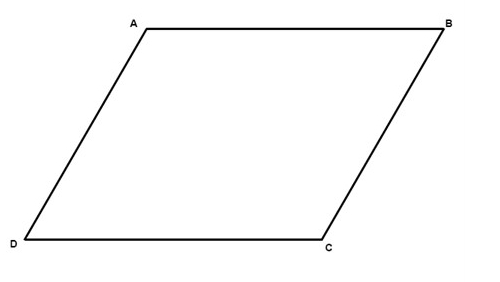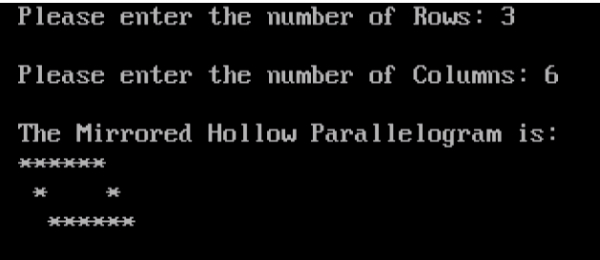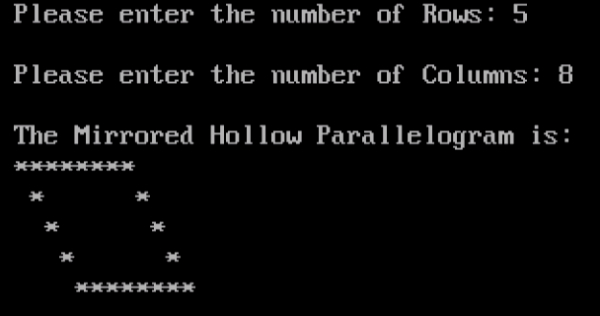# Program to Print Mirrored Hollow Parallelogram in C

CServer Side ProgrammingProgramming

## Program Description

It is a quadrilateral where both pairs of opposite sides are parallel.There are six important properties of parallelograms to know

• Opposite sides are congruent (AB = DC).
• Opposite angels are congruent (D = B).
• Consecutive angles are supplementary (A + D = 180°).
• If one angle is right, then all angles are right.
• The diagonals of a parallelogram bisect each other.
• Each diagonal of a parallelogram separates it into two congruent

## Algorithm

• Accept number of rows and columns from user. Store it in rows and cols variables.
• To iterate though rows, run an outer loop with the loop structure should look like for(r=1; r<=rows; r++).
• To print space, run an inner loop with loop structure for(c=1; c<r; c++). Inside this loop print single blank space.
• Print star to form hollow parallelogram, run another inner loop with the loop structure like for(c=1; c<=cols; c++). Inside this loop, print stars only if r==1 or r==rows or c==1 or c==cols.
• After printing all columns of a row, move to next line i.e. print new line.

## Example

// C program to print mirrored hollow parallelogram
#include <stdio.h>
int main(){
int rows,cols,r,c;
clrscr(); /*Clears the Screen*/
printf("Please enter the number of Rows: ");
scanf("%d", &rows);
printf("\n");
printf("Please enter the number of Columns: ");
scanf("%d", &cols);
printf("\n");
printf("The Mirrored Hollow Parallelogram is: ");
printf("\n");
for(r = 1; r <= rows; r++){
// Display spaces
for(c = 1; c < r; c++) {
printf(" ");
}
// Display hollow parallelogram
for(c = 1; c <= cols; c++) {
if (r == 1 || r == rows || c == 1 || c == cols) {
printf("*");
}
else {
printf(" ");
}
}
printf("\n");
}
getch();
return 0;
}

## Output